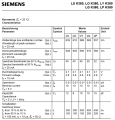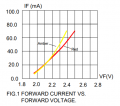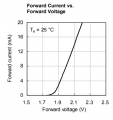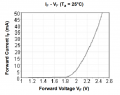# Understanding LED Forward Voltages

#### brightnight1

Joined Jan 13, 2018
91
I understand that if Vforward of an LED is for example 3V, I need at least 3V to turn it on and have current flow. Is there a term in the datasheet for the upper limit of voltage I can use? Can I use 10V or 100V and as long as I limit the current the LED will be fine? I know practically speaking there is limit to what I can use due to arcing, etc., but trying to nail this concept down. Thanks!

#### LowQCab

Joined Nov 6, 2012
2,864
"" Can I use 10V or 100V and as long as I limit the current the LED will be fine? ""
.
Yes.
But, with very high Voltages,
whatever device You use to limit the Current will dissipate a lot of Heat.

100V minus ~3-Volts = 97-Volts,
97-Volts X ~20mA = 1.94-Watts of Heat radiated by a Current-Limiting-Resistor.
.
.
.

#### boostbuck

Joined Oct 5, 2017
273
If you limit the current, the voltage across the LED will be as specified in the datasheet for that item (in your example, approximately 3 volts). It doesn't matter if you supply 10, 100 or 1000V as the current limit will cause the difference between the supply and 3V to appear across the limiter (eg a resistor).

•brightnight1

#### ronsimpson

Joined Oct 7, 2019
2,595
A car light bulb, you put near 12V on it and it lights. The current is not something you think about.
A LED is a current device not a voltage device. You should put 10mA into it. The voltage will be somewhat near the 3V in the data sheet. It is a bad idea to put a voltage on the LED. That is why you see a resistor with a LED. The resistor helps limit the current.

#### WBahn

Joined Mar 31, 2012
28,136
I understand that if Vforward of an LED is for example 3V, I need at least 3V to turn it on and have current flow. Is there a term in the datasheet for the upper limit of voltage I can use? Can I use 10V or 100V and as long as I limit the current the LED will be fine? I know practically speaking there is limit to what I can use due to arcing, etc., but trying to nail this concept down. Thanks!
The data sheet only cares about the voltage actually applied to the LED. If you use a current limiting device, like a resistor, between the LED and the higher supply voltage, then the LED never sees the higher voltage because the current-limiting device is dropping the extra voltage.

Depending on the manufacturer and the specific model of LED in question, the data sheet will usually give a typical and a maximum forward voltage AT a specified test current (and usually at a specified temperature). Occasionally they will also give a minimum, but that's pretty rare. What this information means is that if you pick up an LED (of that model from that manufacturer, though the specs of similar LEDs tend to be similar regardless of source) and put the specified current through it, that the forward voltage you will measure will likely be pretty close to the typical value, but it could be more or less, however it should not be more than the max.

What it does NOT mean is that it is safe to put the maximum voltage across the LED -- that may very well result in the max current being exceeded by a significant amount, even if that particular LED is "typical".

•brightnight1

#### BobTPH

Joined Jun 5, 2013
6,268
The Vf if an LED is always specified at some current. Using a lower voltage will result in less current. Foe example, if the Vf is 20 mA at 3.0V, the LED will still light at 2.9V but will draw less current, maybe 15mA. It will probably put out visible light in a dark room at 2.5V. Somewhere below that, it will cease to put out any visible light.

•bassbindevil and brightnight1

#### WBahn

Joined Mar 31, 2012
28,136
The Vf if an LED is always specified at some current. Using a lower voltage will result in less current. Foe example, if the Vf is 20 mA at 3.0V, the LED will still light at 2.9V but will draw less current, maybe 15mA. It will probably put out visible light in a dark room at 2.5V. Somewhere below that, it will cease to put out any visible light.
But the Vf at the test current is not a specific voltage -- it has a range. So if your LED has a typical Vf of 3.0 V at 20 mA and you take one of those LEDs and put 2.9 V across it, the most likely situation is that it will draw less than 20 mA, but that particular LED might draw more, perhaps considerably more, than 20 mA at that voltage. LED datasheets seldom list a minimum Vf, but let's assume that it is 2.8 V and that the LED you picked is close to that. That means that THAT particular LED will draw 20 mA with a forward voltage of only 2.8 V and that if you apply 2.9 V that it will draw more than 20 mA.

•ronsimpson and BobTPH

#### dl324

Joined Mar 30, 2015
15,510
I understand that if Vforward of an LED is for example 3V, I need at least 3V to turn it on and have current flow.
It will start to emit light before the nominal forward voltage is reached.
Is there a term in the datasheet for the upper limit of voltage I can use? Can I use 10V or 100V and as long as I limit the current the LED will be fine?
We usually think about setting the forward current and let the voltage be whatever it will be (because LEDs from a lot have a range of forward voltages and brightnesses at some current (20mA is a typical figure for standard LEDs)).
I know practically speaking there is limit to what I can use due to arcing, etc., but trying to nail this concept down.
You're not going to get much arcing at 5V or less. Quarter watt resistors will have a working voltage of 200V or so, so no arcing risk there either.

This datasheet happens to give the same forward voltage range for red, orange, yellow, and green:Maximum continuous current is 40mA or 30mA. Peak current is 500mA.This graph shows brightness vs current (down to 0.1mA, except for pure green):Last edited:

Joined Jan 15, 2015
7,134
A quality LED sold by a reputable distributor will include a good data sheet looking like this. Ignore the Absolute Maximum Ratings at Ta= 25°C. Forward Voltage Vf is 2.5 volts @ 20 mA of current. So with 5 volts applied we get 5.0 - 2.5 = 2.5 so we have 2.5 volts @ 20mA So 2.5 / 0.020 Amp = 250 Ohms so you want about a 250 Ohm series resistor. The resistor will drop 2.5 volts @ 20 mA Power is equal to I * E so .020 * 2.5 = 0.05 watt so any 1/4 watt resistor should do fine. Just remember as the voltage drop across the resistor increases so must the rated wattage of the resistor as was pointed out.

Ron

#### WBahn

Joined Mar 31, 2012
28,136
A quality LED sold by a reputable distributor will include a good data sheet looking like this. Ignore the Absolute Maximum Ratings at Ta= 25°C. Forward Voltage Vf is 2.5 volts @ 20 mA of current. So with 5 volts applied we get 5.0 - 2.5 = 2.5 so we have 2.5 volts @ 20mA So 2.5 / 0.020 Amp = 250 Ohms so you want about a 250 Ohm series resistor. The resistor will drop 2.5 volts @ 20 mA Power is equal to I * E so .020 * 2.5 = 0.05 watt so any 1/4 watt resistor should do fine. Just remember as the voltage drop across the resistor increases so must the rated wattage of the resistor as was pointed out.

Ron
Why do you say to ignore the Absolute Max Ratings?

I get that, assuming a Vf of 2.5 V and a 5 V supply, that you would want a 125 Ω current-limiting resistor.

But most of the time the forward voltage at 20 mA in that LED will be closer to 2 V than 2.5 V and some of the time it will be even less than that. So, with a 125 Ω resistor the current would be closer to 24 mA (which exceeds the absolute continuous current rating).

Note that the typical If vs Vf curve in the data sheet is at odds with their tabulated typical values. The curve shows 20 mA at 2.2 V, not the 2.0 V.

#### crutschow

Joined Mar 14, 2008
31,475

Joined Jan 15, 2015
7,134
Why do you say to ignore the Absolute Max Ratings?
Mainly because I don't want someone who is just learning to run a LED at the maximum ratings. Really "ignore" was a poor choice of words. More like I don't recommend it.

Ron

•brightnight1

#### wayneh

Joined Sep 9, 2010
17,201
A practical rule-of-thumb for an LED is that the difference between barely lit and destroyed (due to overcurrent) is about 1/2 volt. The nice chart in #12 from @crutschow shows that. That leaves the problem that @WBahn identified, that the precise voltage range depends on the individual device. It also varies with temperature for an individual LED.

So very precise voltage control can be used to control an LED, if you take the time to start low and determine which voltage gives the current you need.

It's an order of magnitude easier to control the current with, for instance, a resistor.

•brightnight1

#### WBahn

Joined Mar 31, 2012
28,136
A practical rule-of-thumb for an LED is that the difference between barely lit and destroyed (due to overcurrent) is about 1/2 volt. The nice chart in #12 from @crutschow shows that. That leaves the problem that @WBahn identified, that the precise voltage range depends on the individual device. It also varies with temperature for an individual LED.
From a CREE data sheet:This shows a couple of things. For this device, the absolute maximum continuous forward current is 50 mA, which (looking at the Red track) is achieved in a "typical LED" at a forward voltage of about 2.35 V. At 2.5 V, the typical LED has about 70 mA of current, or nearly 50% more than the absolute max rating.

However, this same part lists the Vf for the typical LED at 2.1 V at 20 mA (which agrees with the diagram -- this is not the case with many datasheets) but lists the maximum voltage at this same current as 2.6 V. From the curve, this would result in over 80 mA of current.

Another thing that this curve reveals is that LEDs are actually pretty poor diodes. Once there is much current at all flowing, the voltage vs current curve quickly deviates from the exponential curve one would normally expect for a diode and becomes much closer to being linear as it becomes dominated by essentially resistive effects. This behavior is generally much more pronounced in green and blue LEDs, with red LEDs maintaining the expected exponential behavior better (and yellow/orange LEDs somewhere between). Most generic SPICE LED models don't capture this very well.

Here's another example from a Kingbright data sheet:This is for a green LED with an absolute max continuous forward current of 25 mA and a Vf at 10 mA of 2 V typical and 2.4 V maximum. This is an example of where the data sheet is not self-consistent. This this graph, the typical LED has a current of just 8 mA at 2 V, but this is a relatively small discrepancy compared to some data sheets.

From the multicomp data sheet referred to earlier:

This is a red LED with an asbolute max continuous forward current of 20 mA and a Vf at 20 mA of 2 V typical and 2.5 V max.Here we can see the largely exponential behavior extending to much higher currents, as it common for red LEDs. We also see that at the 2.0 V "typical" Vf that the curve shows only 5 mA (maybe 7 mA) and that 20 mA occurs in the typical LED at a voltage of 2.2 V.

Another thing that is uncommon, but not unheard of, in this data sheet is that the test current is equal to the max absolute current. This underscores an often overlooked point -- the test current is just that, the current at which the manufacturer chose to characterize their devices. Many people infer (and not unreasonably so) that this is the recommended operating point for the device, but that is not always the case. In this case, I suspect that the choice of characterizing it at the max current was so that they could claim the highest value for luminous intensity.

A good rule of thumb is to consider a working maximum continuous design current to be about half the absolute rating. This not only removes most of the life-shorting stress on the part, but it also gives quite a bit more wiggle room to allow for variation device-to-device. The Cree data sheet is explicit about this and state that the recommended drive currents should be between 10 mA and 30 mA (their part has a 50 mA max continuous current rating).

So very precise voltage control can be used to control an LED, if you take the time to start low and determine which voltage gives the current you need.
I don't agree with this since the voltage that gives your the current you need is not a fixed number, even for a specific LED. It is dependent on temperature and, worse, has a negative slope. This means that as the LED heats up, the voltage across it drops at a given current or, equivalently, the current increases at a given voltage. This results in more self-heating, which is a recipe for thermal runaway, particularly in red LEDs since they don't exhibit the resistive-type I-V curve as early as other colors.

It's an order of magnitude easier to control the current with, for instance, a resistor.
Absolutely agree with this -- to the point where I think it is the only rational way to go.

You will see lots of devices where the LED is connected directly to a battery, but this is still using current limiting and not voltage control as it is relying on the relatively high internal resistance of the batteries chosen. If you power those same devices from a "better" battery or a bench supply, it is not uncommon to see then fail very quickly.

#### wayneh

Joined Sep 9, 2010
17,201
I don't agree with this since the voltage that gives your the current you need is not a fixed number, even for a specific LED. It is dependent on temperature and, worse, has a negative slope. This means that as the LED heats up, the voltage across it drops at a given current or, equivalently, the current increases at a given voltage.
Have you ever tried controlling LED brightness with voltage? It works just fine. Of course you need a well-regulated supply that can hold the voltage (much) better than ±0.1V.

Thermal runaway might be a risk if you operate near max current but at currents less than that, it just doesn't happen.

#### WBahn

Joined Mar 31, 2012
28,136
Have you ever tried controlling LED brightness with voltage? It works just fine. Of course you need a well-regulated supply that can hold the voltage (much) better than ±0.1V.

Thermal runaway might be a risk if you operate near max current but at currents less than that, it just doesn't happen.
If you keep the power dissipation low enough so that ambient cooling responds strongly enough to compensate for the increase power as it heats, then this is likely sufficient at a temperature equilibrium can be established at a low enough temperature to stabilize it. The resistive-nature of the I-V curve also helps in this regard.

But I see no practical reason to do so (and am interested in hearing of ones that you know of), except when characterizing the device. Though even then I suspect a current-source is probably used.

#### MrChips

Joined Oct 2, 2009
28,093
Let us use as an example of an LED whose If = 20mA at Vf = 2V.

The static resistance is 2V/20mA = 100Ω
The dynamic resistance, ΔV/ΔI is much lower, about 10Ω.

A supply voltage of 4V with a 100Ω series resistor is going stabilize the LED current to about 20mA even if Vf differs from 2V.

#### WBahn

Joined Mar 31, 2012
28,136
Let us use as an example of an LED whose If = 20mA at Vf = 2V.

The static resistance is 2V/20mA = 100Ω
The dynamic resistance, ΔV/ΔI is much lower, about 10Ω.

A supply voltage of 4V with a 100Ω series resistor is going stabilize the LED current to about 20mA even if Vf differs from 2V.
Sure, which is why @wayneh and I both strongly recommend external control of the current as opposed to trying to control it applying a voltage directly.

Note that, in your example, the current can vary quite a bit because of the very limited overhead voltage. If the Vf of the LED is actually 2.5 V, the current would be reduced by about 25%. That's a fairly significant change, though fortunately (in most applications), the difference perceived in the LED output would be minimal.

#### MrChips

Joined Oct 2, 2009
28,093
Sure, which is why @wayneh and I both strongly recommend external control of the current as opposed to trying to control it applying a voltage directly.

Note that, in your example, the current can vary quite a bit because of the very limited overhead voltage. If the Vf of the LED is actually 2.5 V, the current would be reduced by about 25%. That's a fairly significant change, though fortunately (in most applications), the difference perceived in the LED output would be minimal.
My point is, the supply voltage should be at minimum twice Vf, the higher the better.
Then you can adjust for LED brightness by varying either the series resistance or the supply voltage. And you can ignore both If and Vf.